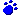I have changed many of the links and added new games for you.  If a link doesn't work please email me.Mad Math Minutes Mrs. Stein's Math Calculator Magic Squares Math Quiz Room 108 Math Rick's Math Web Interactive Math Activites Math Flash Cards Spacey Math MATH ALL AROUND US! Math Brain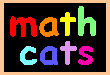Drill Games Math General Money Math Time Math Numeracy Aunty Math Multiplication Table Applet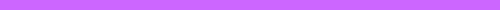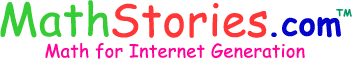Addition Sums 10-18First Grade SkillsSecond Grade Skill BuildersThird Grade Skill BuildersAddition-2 digit numbers
 Online Math Flash Cards Times Tables Fraction Addition

Brain Benders
Long Division
ThingsMath StoriesAutoMathsBase Ten Count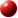Number Sense GamesMeasurement GamesMath Fact CafeKids NumbersMath for KidsMath Playground Interactive Number Games ISplat Number SquaresFind the NumbersFind the Target NumberFind 11 More than the Target NumberNumber Square AdditionPlace Value